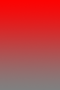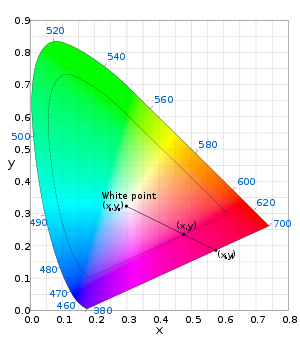# Colorfulness

Colorfulness

In colorimetry and color theory, colorfulness, chroma, and saturation are related but distinct concepts referring to the perceived intensity of a specific color. Colorfulness is the degree of difference between a color and gray. Chroma is the colorfulness relative to the brightness of another color that appears white under similar viewing conditions. Saturation is the colorfulness of a color relative to its own brightness. Though this general concept is intuitive, terms such as chroma, saturation, purity, and intensity are often used without great precision, and even when well-defined depend greatly on the specific color model in use.

A highly colorful stimulus is vivid and intense, while a less colorful stimulus appears more muted, closer to gray. With no colorfulness at all, a color is a “neutral” gray (an image with no colorfulness in any of its colors is called grayscale). With three attributes—colorfulness (or chroma or saturation), lightness (or brightness), and hue—any color can be described.

## SaturationScale of saturation (0% at bottom and its black and white).

Saturation is one of three coordinates in the HSL and HSV color spaces. Note that virtually all computer software implementing these spaces use a very rough approximation to calculate the value they call "saturation", such as the formula described for HSV and this value has little, if anything, to do with the description shown here.

The saturation of a color is determined by a combination of light intensity and how much it is distributed across the spectrum of different wavelengths. The purest (most saturated) color is achieved by using just one wavelength at a high intensity, such as in laser light. If the intensity drops, then as a result the saturation drops. To desaturate a color of given intensity in a subtractive system (such as watercolor), one can add white, black, gray, or the hue's complement.

Various correlates of saturation follow.

CIELUV
The chroma normalized by the lightness:$s_{uv}=\frac{C^*_{uv}}{L^*}=13 \sqrt{(u'-u'_n)^2+(v'-v'_n)^2}$

where (un, vn) is the chromaticity of the white point, and chroma is defined below.

By analogy, in CIELAB this would yield:$s_{ab}=\frac{C^*_{ab}}{L^*}=\frac{\sqrt{{a^*}^2+{b^*}^2}}{L^*}$

The CIE has not formally recommended this equation since CIELAB has no chromaticity diagram, and this definition therefore lacks direct correlation with older concepts of saturation. Nevertheless, this equation provides a reasonable predictor of saturation, and demonstrates that adjusting the lightness in CIELAB while holding (a*, b*) fixed does affect the saturation.

But the following formula is in agreement with the human perception of saturation: The formula proposed by Eva Lübbe is in agreement with the verbal definition of Manfred Richter: Saturation is the proportion of pure chromatic color in the total color sensation.$S_{ab}=\frac{C^*_{ab}}{\sqrt{{C^*_{ab} }^2+{L^*}^2}} 100 %$

where Sab is the saturation, L* the lightness and C*ab is the chroma of the color.

CIECAM02
The square root of the colorfulness divided by the brightness:$s=\sqrt{M/Q}$

This definition is inspired by experimental work done with the intention of remedying CIECAM97s's poor performance. M is proportional to the chroma C (M = CFL0.25), thus the CIECAM02 definition bears some similarity to the CIELUV definition. An important difference is that the CIECAM02 model accounts for the viewing conditions through the parameter FL.

## Excitation purityExcitation purity is the relative distance from the white point. Contours of constant purity can be found by shrinking the spectral locus about the white point. The points along the line segment have the same hue, with pe increasing from 0 to 1 between the white point and position on the spectral locus (position of the color on the horseshoe shape in the diagram) or (as at the saturated end of the line shown in the diagram) position on the line of purples.

The excitation purity (purity for short) of a stimulus is its difference from the illuminant's white point relative to the furthest point on the chromaticity diagram with the same hue (dominant wavelength for monochromatic sources); using the CIE 1931 color space:$p_e = \sqrt{\frac{(x - x_n)^2 + (y - y_n)^2}{(x_I - x_n)^2 + (y_I - y_n)^2}}$

where (xn, yn) is the chromaticity of the white point and (xI, yI) is the point on the perimeter whose line segment to the white point contains the chromaticity of the stimulus. Different color spaces, such as CIELAB or CIELUV may be used, and will yield different results.

## Chroma in CIE 1976 L*a*b* and L*u*v* color spaces

The naïve definition of saturation does not specify its response function. In the CIE XYZ and RGB color spaces, the saturation is defined in terms of additive color mixing, and has the property of being proportional to any scaling centered at white or the white point illuminant. However, both color spaces are nonlinear in terms of psychovisually perceived color differences. It is also possible, and sometimes desirable to define a saturation-like quantity that is linearized in term of the psychovisual perception.

In the CIE 1976 L*a*b* and L*u*v* color spaces, the unnormalized chroma is the radial component of the cylindrical coordinate CIE L*C*h (lightness, chroma, hue) representation of the L*a*b* and L*u*v* color spaces, also denoted as CIE L*C*h(a*b*)[citation needed] or CIE L*C*h for short, and CIE L*C*h(u*v*). The transformation of (a*, b*) to (C*ab, hab) is given by:$C_{ab}^* = \sqrt{a^{*2} + b^{*2}}$$h_{ab} = \arctan \frac{b^{*}}{a^{*}}$

and analogously for CIE L*C*h(u*v*).

The chroma in the CIE L*C*h(a*b*) and CIE L*C*h(u*v*) coordinates has the advantage of being more psychovisually linear, yet they are non-linear in terms of linear component color mixing. And therefore, chroma in CIE 1976 L*a*b* and L*u*v* color spaces is very much different from the traditional sense of "saturation".

### Chroma in color appearance models

Another, psychovisually even more accurate, but also more complex method to obtain or specify the saturation is to use the color appearance model, like CIECAM. The chroma component of the LCh (lightness, chroma, hue) coordinate, and becomes a function of parameters like the chrominance and physical brightness of the illumination, or the characteristics of the emitting/reflecting surface, which is also psychovisually more sensible.

Wikimedia Foundation. 2010.

### Look at other dictionaries:

• colorfulness — (Amer.) n. being full of color; being richly descriptive …   English contemporary dictionary

• colorfulness — noun see colorful …   New Collegiate Dictionary

• colorfulness — See colorfully. * * * …   Universalium

• colorfulness — noun the state of being colorful …   Wiktionary

• colorfulness — col·or·ful·ness …   English syllables

• colorfulness — noun see colorful …   Useful english dictionary

• HSL and HSV — Fig. 1. HSL (a–d) and HSV (e–h). Above (a, e): cut away 3D models of each. Below: two dimensional plots showing two of a model’s three parameters at once, holding the other constant: cylindrical shells (b, f) of constant saturation, in this case… …   Wikipedia

• CIECAM02 — ist ein Farberscheinungsmodell (engl. Color Appearance Model) von der internationalen Beleuchtungskommission CIE (commission internationale de l éclairage) und der Nachfolger von CIECAM97. Es basiert auf dem CIECAM97 Modell und beinhaltet einige… …   Deutsch Wikipedia

• Color — This article is about the perceptual property. For other uses, see Color (disambiguation). For usage of color on Wikipedia, see Wikipedia:Colors. Colored pencils Color or colour (see spelling differences) is the visual perceptual property… …   Wikipedia

• CIECAM02 — Published in 2002 by the CIE Technical Committee 8 01 ( Colour Appearance Modelling for Color Management Systems ), as of 2008 CIECAM02 is the most recent color appearance model ratified by the CIE, and the successor of CIECAM97s.cite… …   Wikipedia